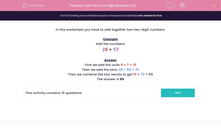# Add Two Two-Digit Numbers (2)

In this worksheet, students add together two-digit numbers by adding the units, then adding the tens and combining the results.Key stage:  KS 2

Curriculum topic:   Maths and Numerical Reasoning

Curriculum subtopic:   Mixed Problems

Difficulty level:#### Worksheet Overview

In this worksheet you have to add together two two-digit numbers.

Example

28 + 57

First we add the units 8 + 7 = 15

Then we add the tens, 20 + 50 = 70

Then we combine the two results to get 15 70 = 85